Publicité

# Wave mechanics, 8(4)

Assistant Professor Chemical Engineering, Chemistry à Memorial University of Newfoundland
31 Mar 2020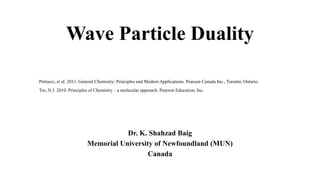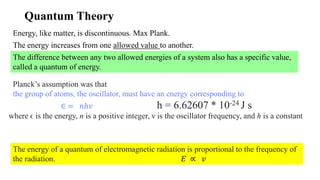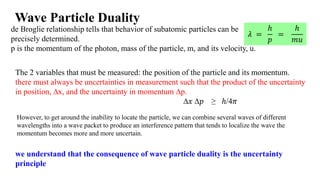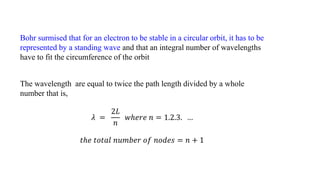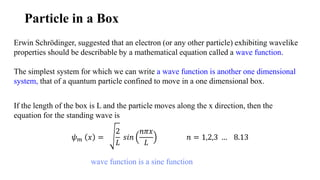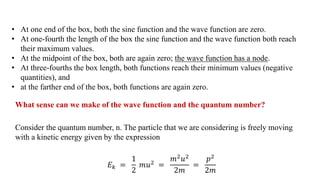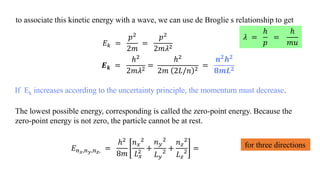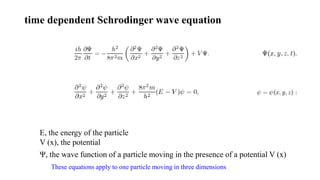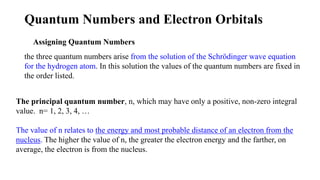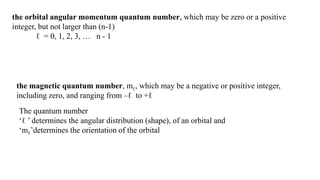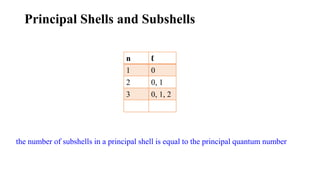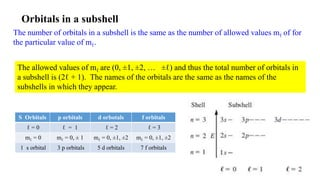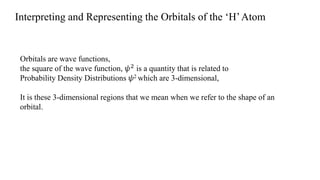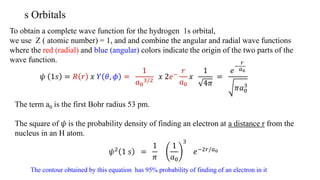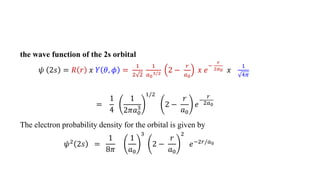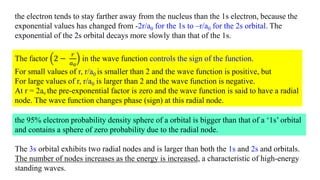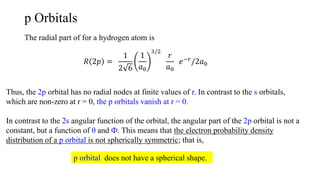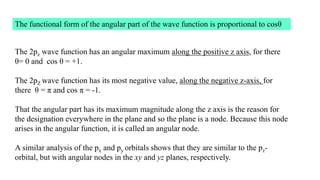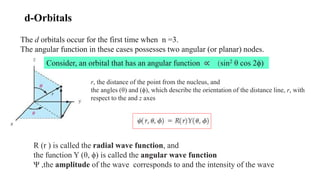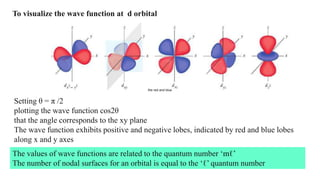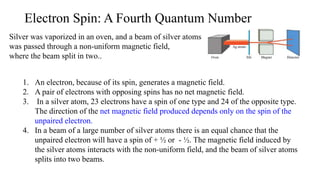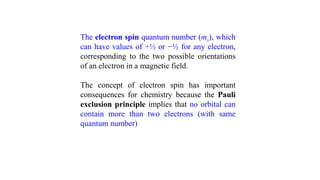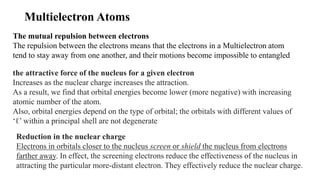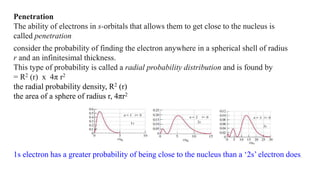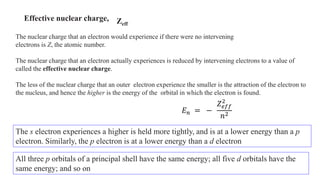1 sur 25
Publicité

### Wave mechanics, 8(4)

1. Wave Particle Duality Dr. K. Shahzad Baig Memorial University of Newfoundland (MUN) Canada Petrucci, et al. 2011. General Chemistry: Principles and Modern Applications. Pearson Canada Inc., Toronto, Ontario. Tro, N.J. 2010. Principles of Chemistry. : a molecular approach. Pearson Education, Inc.
2. Quantum Theory The energy increases from one allowed value to another. Energy, like matter, is discontinuous. Max Plank. The difference between any two allowed energies of a system also has a specific value, called a quantum of energy. Planck’s assumption was that the group of atoms, the oscillator, must have an energy corresponding to ∈ = 𝑛ℎ𝑣 h = 6.62607 * 10-24 J s where ϵ is the energy, n is a positive integer, ν is the oscillator frequency, and h is a constant The energy of a quantum of electromagnetic radiation is proportional to the frequency of the radiation. 𝐸 ∝ 𝑣
3. we understand that the consequence of wave particle duality is the uncertainty principle Wave Particle Duality de Broglie relationship tells that behavior of subatomic particles can be precisely determined. p is the momentum of the photon, mass of the particle, m, and its velocity, u. 𝜆 = ℎ 𝑝 = ℎ 𝑚𝑢 However, to get around the inability to locate the particle, we can combine several waves of different wavelengths into a wave packet to produce an interference pattern that tends to localize the wave the momentum becomes more and more uncertain. The 2 variables that must be measured: the position of the particle and its momentum. there must always be uncertainties in measurement such that the product of the uncertainty in position, ∆x, and the uncertainty in momentum ∆p. ∆𝑥 ∆𝑝 ≥ ℎ/4𝜋
4. The wavelength are equal to twice the path length divided by a whole number that is, 𝜆 = 2𝐿 𝑛 𝑤ℎ𝑒𝑟𝑒 𝑛 = 1.2.3. … 𝑡ℎ𝑒 𝑡𝑜𝑡𝑎𝑙 𝑛𝑢𝑚𝑏𝑒𝑟 𝑜𝑓 𝑛𝑜𝑑𝑒𝑠 = 𝑛 + 1 Bohr surmised that for an electron to be stable in a circular orbit, it has to be represented by a standing wave and that an integral number of wavelengths have to fit the circumference of the orbit
5. Particle in a Box Erwin Schrödinger, suggested that an electron (or any other particle) exhibiting wavelike properties should be describable by a mathematical equation called a wave function. The simplest system for which we can write a wave function is another one dimensional system, that of a quantum particle confined to move in a one dimensional box. If the length of the box is L and the particle moves along the x direction, then the equation for the standing wave is 𝜓 𝑚 𝑥 = 2 𝐿 𝑠𝑖𝑛 𝑛𝜋𝑥 𝐿 𝑛 = 1,2,3 … 8.13 wave function is a sine function
6. • At one end of the box, both the sine function and the wave function are zero. • At one-fourth the length of the box the sine function and the wave function both reach their maximum values. • At the midpoint of the box, both are again zero; the wave function has a node. • At three-fourths the box length, both functions reach their minimum values (negative quantities), and • at the farther end of the box, both functions are again zero. What sense can we make of the wave function and the quantum number? Consider the quantum number, n. The particle that we are considering is freely moving with a kinetic energy given by the expression 𝐸 𝑘 = 1 2 𝑚𝑢2 = 𝑚2 𝑢2 2𝑚 = 𝑝2 2𝑚
7. to associate this kinetic energy with a wave, we can use de Broglie s relationship to get 𝐸 𝑘 = 𝑝2 2𝑚 = 𝑝2 2𝑚𝜆2 𝑬 𝒌 = ℎ2 2𝑚𝜆2 = ℎ2 2𝑚 2𝐿/𝑛 2 = 𝒏 𝟐 𝒉 𝟐 𝟖𝒎𝑳 𝟐 The lowest possible energy, corresponding is called the zero-point energy. Because the zero-point energy is not zero, the particle cannot be at rest. 𝐸 𝑛 𝑥,𝑛 𝑦,𝑛 𝑧, = ℎ2 8𝑚 𝑛 𝑥 2 𝐿 𝑥 2 + 𝑛 𝑦 2 𝐿 𝑦 2 + 𝑛 𝑧 2 𝐿 𝑧 2 = for three directions 𝜆 = ℎ 𝑝 = ℎ 𝑚𝑢 If Ek increases according to the uncertainty principle, the momentum must decrease.
8. E, the energy of the particle V (x), the potential Ψ, the wave function of a particle moving in the presence of a potential V (x) time dependent Schrodinger wave equation These equations apply to one particle moving in three dimensions
9. Quantum Numbers and Electron Orbitals Assigning Quantum Numbers the three quantum numbers arise from the solution of the Schrödinger wave equation for the hydrogen atom. In this solution the values of the quantum numbers are fixed in the order listed. The principal quantum number, n, which may have only a positive, non-zero integral value. n= 1, 2, 3, 4, … The value of n relates to the energy and most probable distance of an electron from the nucleus. The higher the value of n, the greater the electron energy and the farther, on average, the electron is from the nucleus.
10. the magnetic quantum number, mℓ, which may be a negative or positive integer, including zero, and ranging from –ℓ to +ℓ The quantum number ‘ℓ ’ determines the angular distribution (shape), of an orbital and ‘mℓ’determines the orientation of the orbital the orbital angular momentum quantum number, which may be zero or a positive integer, but not larger than (n-1) ℓ = 0, 1, 2, 3, … n - 1
11. Principal Shells and Subshells the number of subshells in a principal shell is equal to the principal quantum number n ℓ 1 0 2 0, 1 3 0, 1, 2
12. The number of orbitals in a subshell is the same as the number of allowed values mℓ of for the particular value of mℓ. The allowed values of mℓ are (0, ±1, ±2, … ±ℓ) and thus the total number of orbitals in a subshell is (2ℓ + 1). The names of the orbitals are the same as the names of the subshells in which they appear. S Orbitals p orbitals d orbotals f orbitals ℓ = 0 ℓ = 1 ℓ = 2 ℓ = 3 mℓ = 0 mℓ = 0, ± 1 mℓ = 0, ±1, ±2 mℓ = 0, ±1, ±2 1 s orbital 3 p orbitals 5 d orbitals 7 f orbitals Orbitals in a subshell
13. Interpreting and Representing the Orbitals of the ‘H’Atom Orbitals are wave functions, the square of the wave function, 𝜓2 is a quantity that is related to Probability Density Distributions 𝜓2 which are 3-dimensional, It is these 3-dimensional regions that we mean when we refer to the shape of an orbital.
14. s Orbitals 𝜓 1𝑠 = 𝑅 𝑟 𝑥 𝑌 𝜃, 𝜙 = 1 𝑎0 3/2 𝑥 2𝑒− 𝑟 𝑎0 𝑥 1 4𝜋 = 𝑒 − 𝑟 𝑎0 𝜋𝑎0 3 To obtain a complete wave function for the hydrogen 1s orbital, we use Z ( atomic number) = 1, and and combine the angular and radial wave functions where the red (radial) and blue (angular) colors indicate the origin of the two parts of the wave function. The term a0 is the first Bohr radius 53 pm. The square of 𝜓 is the probability density of finding an electron at a distance r from the nucleus in an H atom. 𝜓2 1 𝑠 = 1 𝜋 1 𝑎0 3 𝑒−2𝑟/𝑎0 The contour obtained by this equation has 95% probability of finding of an electron in it
15. the wave function of the 2s orbital 𝜓 2𝑠 = 𝑅 𝑟 𝑥 𝑌 𝜃, 𝜙 = 1 2 2 1 𝑎0 3/2 2 − 𝑟 𝑎0 𝑥 𝑒 − 𝑟 2𝑎0 𝑥 1 4𝜋 = 1 4 1 2𝜋𝑎0 3 1/2 2 − 𝑟 𝑎0 𝑒 − 𝑟 2𝑎0 𝜓2 2𝑠 = 1 8𝜋 1 𝑎0 3 2 − 𝑟 𝑎0 2 𝑒−2𝑟/𝑎0 The electron probability density for the orbital is given by
16. the electron tends to stay farther away from the nucleus than the 1s electron, because the exponential values has changed from -2r/a0 for the 1s to –r/a0 for the 2s orbital. The exponential of the 2s orbital decays more slowly than that of the 1s. The factor 2 − 𝑟 𝑎0 in the wave function controls the sign of the function. For small values of r, r/a0 is smaller than 2 and the wave function is positive, but For large values of r, r/a0 is larger than 2 and the wave function is negative. At r = 2a0 the pre-exponential factor is zero and the wave function is said to have a radial node. The wave function changes phase (sign) at this radial node. The 3s orbital exhibits two radial nodes and is larger than both the 1s and 2s and orbitals. The number of nodes increases as the energy is increased, a characteristic of high-energy standing waves. the 95% electron probability density sphere of a orbital is bigger than that of a ‘1s’ orbital and contains a sphere of zero probability due to the radial node.
17. p Orbitals The radial part of for a hydrogen atom is 𝑅 2𝑝 = 1 2 6 1 𝑎0 3/2 𝑟 𝑎0 𝑒−𝑟 /2𝑎0 Thus, the 2p orbital has no radial nodes at finite values of r. In contrast to the s orbitals, which are non-zero at r = 0, the p orbitals vanish at r = 0. In contrast to the 2s angular function of the orbital, the angular part of the 2p orbital is not a constant, but a function of θ and Ф. This means that the electron probability density distribution of a p orbital is not spherically symmetric; that is, p orbital does not have a spherical shape.
18. The 2pz wave function has an angular maximum along the positive z axis, for there θ= 0 and cos θ = +1. The 2pZ wave function has its most negative value, along the negative z-axis, for there θ = π and cos π = -1. That the angular part has its maximum magnitude along the z axis is the reason for the designation everywhere in the plane and so the plane is a node. Because this node arises in the angular function, it is called an angular node. A similar analysis of the px and py orbitals shows that they are similar to the pz- orbital, but with angular nodes in the xy and yz planes, respectively. The functional form of the angular part of the wave function is proportional to cosθ
19. d-Orbitals The d orbitals occur for the first time when n =3. The angular function in these cases possesses two angular (or planar) nodes. r, the distance of the point from the nucleus, and the angles (θ) and (ϕ), which describe the orientation of the distance line, r, with respect to the and z axes R (r ) is called the radial wave function, and the function Y (θ, ϕ) is called the angular wave function Ψ ,the amplitude of the wave corresponds to and the intensity of the wave Consider, an orbital that has an angular function ∝ (sin2 θ cos 2ϕ)
20. Setting θ = π /2 plotting the wave function cos2θ that the angle corresponds to the xy plane The wave function exhibits positive and negative lobes, indicated by red and blue lobes along x and y axes To visualize the wave function at d orbital the red and blue The values of wave functions are related to the quantum number ‘mℓ’ The number of nodal surfaces for an orbital is equal to the ‘ℓ’ quantum number
21. Electron Spin: A Fourth Quantum Number Silver was vaporized in an oven, and a beam of silver atoms was passed through a non-uniform magnetic field, where the beam split in two.. 1. An electron, because of its spin, generates a magnetic field. 2. A pair of electrons with opposing spins has no net magnetic field. 3. In a silver atom, 23 electrons have a spin of one type and 24 of the opposite type. The direction of the net magnetic field produced depends only on the spin of the unpaired electron. 4. In a beam of a large number of silver atoms there is an equal chance that the unpaired electron will have a spin of + ½ or - ½. The magnetic field induced by the silver atoms interacts with the non-uniform field, and the beam of silver atoms splits into two beams.
22. The electron spin quantum number (ms), which can have values of +½ or −½ for any electron, corresponding to the two possible orientations of an electron in a magnetic field. The concept of electron spin has important consequences for chemistry because the Pauli exclusion principle implies that no orbital can contain more than two electrons (with same quantum number)
23. Multielectron Atoms The mutual repulsion between electrons The repulsion between the electrons means that the electrons in a Multielectron atom tend to stay away from one another, and their motions become impossible to entangled the attractive force of the nucleus for a given electron Increases as the nuclear charge increases the attraction. As a result, we find that orbital energies become lower (more negative) with increasing atomic number of the atom. Also, orbital energies depend on the type of orbital; the orbitals with different values of ‘ℓ’ within a principal shell are not degenerate. Reduction in the nuclear charge Electrons in orbitals closer to the nucleus screen or shield the nucleus from electrons farther away. In effect, the screening electrons reduce the effectiveness of the nucleus in attracting the particular more-distant electron. They effectively reduce the nuclear charge.
24. Penetration The ability of electrons in s-orbitals that allows them to get close to the nucleus is called penetration. consider the probability of finding the electron anywhere in a spherical shell of radius r and an infinitesimal thickness. This type of probability is called a radial probability distribution and is found by = R2 (r) x 4π r2 the radial probability density, R2 (r) the area of a sphere of radius r, 4πr2 1s electron has a greater probability of being close to the nucleus than a ‘2s’ electron does,
25. Effective nuclear charge, The nuclear charge that an electron would experience if there were no intervening electrons is Z, the atomic number. The nuclear charge that an electron actually experiences is reduced by intervening electrons to a value of called the effective nuclear charge. The less of the nuclear charge that an outer electron experience the smaller is the attraction of the electron to the nucleus, and hence the higher is the energy of the orbital in which the electron is found. Zeff The s electron experiences a higher is held more tightly, and is at a lower energy than a p electron. Similarly, the p electron is at a lower energy than a d electron All three p orbitals of a principal shell have the same energy; all five d orbitals have the same energy; and so on 𝐸 𝑛 = − 𝑍 𝑒𝑓𝑓 2 𝑛2

### Notes de l'éditeur

1. Classical theory predicts that the intensity of the radiation emitted would increase indefinitely, Allowed value : discrete set of value of specific value = Objects emit radiation at all temperatures, not just at high temperatures. For example, night-vision goggles makes infrared radiation emitted by objects visible in the dark. A red-hot object has a spectrum that peaks around 675 nm, 𝐸 = ℎ𝑣 : the higher the frequency, the greater the energy
2. In the previous section, we examined some successes of the Bohr theory and pointed out its inability to deal with multielectron atoms. A decade or so after Bohr s work on hydrogen, two landmark ideas stimulated a new approach to quantum mechanics. Those ideas are considered in this section and the new quantum mechanics wave mechanics in the next.
3. sin 0 = 0, sin 𝜋 / 2 = 1, sin 𝜋 = 0 , sin 3𝜋 / 2 = -1
4. where there is one quantum number for each dimension. Thus, a three dimensional system needs three quantum numbers. With these particle-in-abox ideas, we can now discuss solving the quantum mechanical problem of the hydrogen atom. Zero-point energy (ZPE) is the lowest possible energy that a quantum mechanical system may have. there is a finite, minimum amount of motion (more accurately, kinetic energy) in all matter, even at absolute zero. For example, chemical bonds continue to vibrate in predictable ways
5. Newton’s equation F = ma describes the dynamics of a particle in classical physics. However, there is an important difference. By solving Newton’s equation we can determine the position of a particle as a function of time, whereas by solving Schr¨odinger’s equation, what we get is a wave function Ψ(x,t) which tells us (after we square the wave function) how the probability of finding the particle in some region in space varies as a function of time In some situations the potential energy does not depend on time $t.$ In this case we can often solve the problem by considering the simpler time-independent version of the Schrödinger equation for a function $\psi$ depending only on space,
6. by specifying three quantum numbers in a wave function we obtain an orbital. Here, we explore the combinations of quantum numbers that produce different orbitals.
7. to describe the three-dimensional probability density distributions obtained for the various orbitals in the hydrogen atom.
8. radial wave function R(r) and the angular wave function Y ( 𝜃, 𝜙
9. the p orbitals vanish at r = 0. This difference will have an important consequence when we consider multi-electron atoms.
10. The p orbitals are usually represented as directed along the perpendicular x, y, and z axes, and the symbols , px py pz and are often used. The pz orbital has ml = 0. z The situation with px, py is more complex, however. Each of these orbitals has contributions from both mℓ= -1. ; mℓ= 1 and
11. The coordinates x, y, and z are expressed in terms of the distance r and the angles and f. u
12. The orbital having same energy are the degenerate orbitals. eg the three subshells of p orbital are px, py and pz. When they are not bonding with other atomic orbital then they are degenerate. In case of s2p1 electronic configuration the one electron of p can stay in px or py or pz because energetically they are same. But when it will take part in bonding then one p subshell will be of different from other two. then this will not be degenerate with other two.
13. the energy of an orbital is given by the proportionality
Publicité